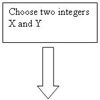#### You may also like### Route to Root

A sequence of numbers x1, x2, x3, ... starts with x1 = 2, and, if you know any term xn, you can find the next term xn+1 using the formula: xn+1 = (xn + 3/xn)/2 . Calculate the first six terms of this sequence. What do you notice? Calculate a few more terms and find the squares of the terms. Can you prove that the special property you notice about this sequence will apply to all the later terms of the sequence? Write down a formula to give an approximation to the cube root of a number and test it for the cube root of 3 and the cube root of 8. How many terms of the sequence do you have to take before you get the cube root of 8 correct to as many decimal places as your calculator will give? What happens when you try this method for fourth roots or fifth roots etc.?### Divided Differences

When in 1821 Charles Babbage invented the `Difference Engine' it was intended to take over the work of making mathematical tables by the techniques described in this article.### Procedure Solver

Can you think like a computer and work out what this flow diagram does?

# Semicircle

##### Age 16 to 18Challenge Level

Have you managed to solve the entire Stage 5 Cipher Challenge? Solutions are now closed, but perhaps you want to take up the full challenge.

Successful solvers of this part were:

Mahdokht from Farzanegan of Kermanshah
Patrick from Woodbridge School, England
An Anonymous Solver from Somewhere in the US
Joseph from Hong Kong

The solution is:

If you can read this, well done! This sort of cipher is called a polyalphabetic cipher. For examples like this one with a keyword of length two, it's possible to solve it by hand just trying possibilities. However, with a longer keyword this gets very hard. There are more sophisticated techniques, one of which is called Kasiski examination. This involves looking for repeated letters in the ciphertext. It's likely that the number of letters inbetween these repeats is a multiple of the keyword length. Look at wikipedia for more information! By the way, if you didn't work it out, the previous cipher was a keyword substitution cipher with keyword keyword. So far, we've only considered substitution ciphers, but there are other alternatives. We could for example reorder the ciphertext.

vigenere keyword: pi